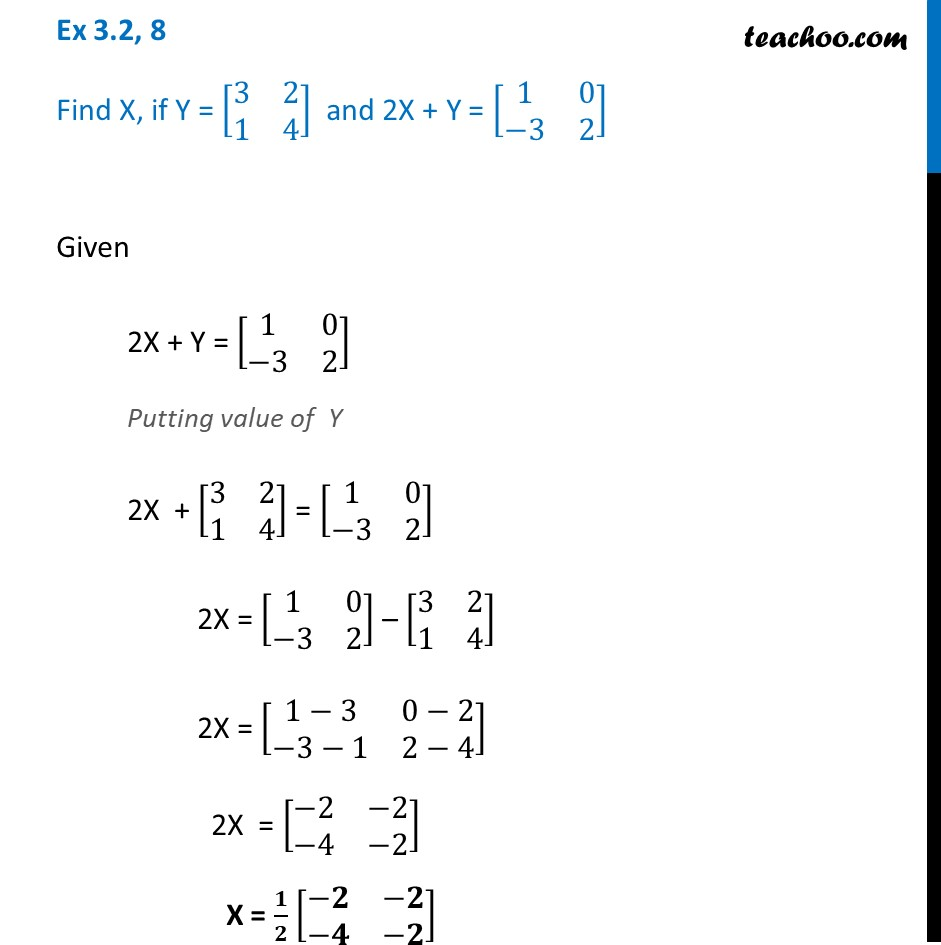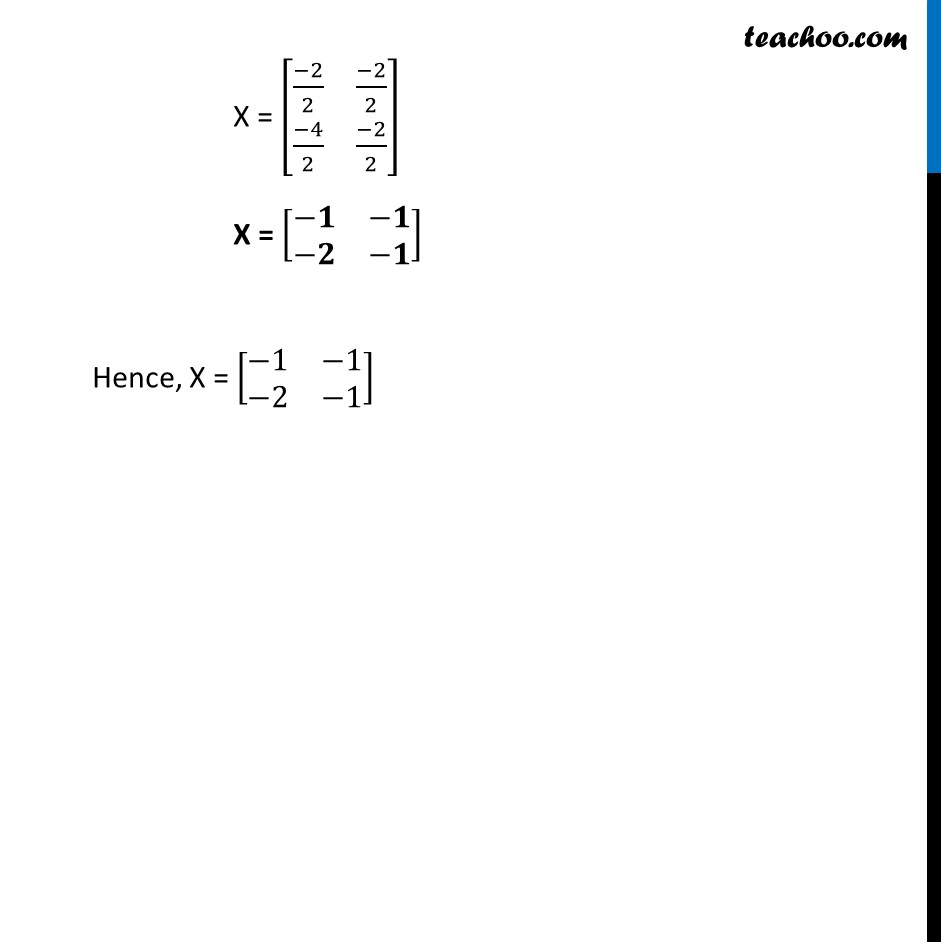Chapter 3 Class 12 Matrices
Concept wiseLearn in your speed, with individual attention - Teachoo Maths 1-on-1 Class

### Transcript

Ex 3.2, 8 Find X, if Y = [■8(3&2@1&4)] and 2X + Y = [■8(1&0@−3&2)] Given 2X + Y = [■8(1&0@−3&2)] Putting value of Y 2X + [■8(3&2@1&4)] = [■8(1&0@−3&2)] 2X = [■8(1&0@−3&2)] – [■8(3&2@1&4)] 2X = [■8(1−3&0−2@−3−1&2−4)] 2X = [■8(−2&−2@−4&−2)] X = 𝟏/𝟐 [■8(−𝟐&−𝟐@−𝟒&−𝟐)] X = [■8((−2)/2&(−2)/2@(−4)/2&(−2)/2)] X = [■8(−𝟏&−𝟏@−𝟐&−𝟏)] Hence, X = [■8(−1&−1@−2&−1)]